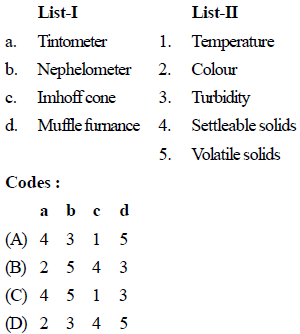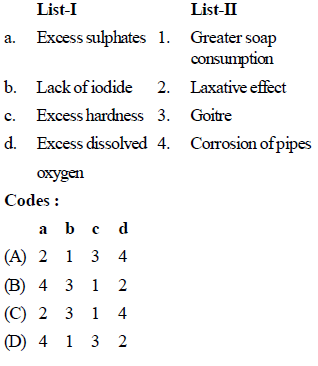# Test: Environment Engineering Practices- 2

## 25 Questions MCQ Test Civil Engineering SSC JE (Technical) | Test: Environment Engineering Practices- 2

Description
Attempt Test: Environment Engineering Practices- 2 | 25 questions in 50 minutes | Mock test for Civil Engineering (CE) preparation | Free important questions MCQ to study Civil Engineering SSC JE (Technical) for Civil Engineering (CE) Exam | Download free PDF with solutions
QUESTION: 1

Solution:
QUESTION: 2

Solution:
QUESTION: 3

### Which is the best sewer material to resist hydrogen sulphide corrosion ?

Solution:
QUESTION: 4

A commonly used handpump is the

Solution:
QUESTION: 5

The standard turbidity produced by one mg of silicon dioxide (silica) in one litre of distilled water, is called

Solution:
QUESTION: 6

Pick up the correct statement(s)

Solution:
QUESTION: 7

One True Colour Unit (TCU) is the colour produced by

Solution:
QUESTION: 8

The threshold odour number (TON) for a water sample of 40 ml, dilute to standard 200 ml mixture, in which odour is just barely detectable to the sense of smell, is

Solution:

Threshold odour number represent the dilution ratio at which the odour is hardly detectable.
T.O.N. = 200/40 = 5

QUESTION: 9

Water is considered ‘hard’, if its hardness is of the order of

Solution:
QUESTION: 10

Higher quantities of copper, more than 2.5 mg/l or so, may cause diseases pertaining to

Solution:
QUESTION: 11

The maximum allowable concentration of iron in water is

Solution:
QUESTION: 12

The commonly used indicator for measuring iron concentration in water is

Solution:
QUESTION: 13

The maximum safe permissible limit of chlorides in domestic water supplies is

Solution:
QUESTION: 14

Breweries and distilleries preferably require

Solution:
QUESTION: 15

Electrical conductivity (EC) of water and total dissolved solids (TDS) are interrelated. The value of EC will

Solution:
QUESTION: 16

In which one of the following tests is the organic matter in the waste mater used as food by micro-organisms?

Solution:
QUESTION: 17

Which one of the following organisms is responsible for enteric fever ?

Solution:
QUESTION: 18

Presence of nitrogen in a waste water sample is due to the decomposition of

Solution:
QUESTION: 19

Which of the following cations impart(s) pseudohardness to water ?

Solution:
QUESTION: 20

Match List-I (Equipment) with List-II (Parameter) and select the correct answer using the codes given below the lits :Solution:
QUESTION: 21

What is the most common cause of acidity in water ?

Solution:
QUESTION: 22

Match List-I (Parameter) with List-Ii (Impact) and select the correct answer using the codes given below the lists :Solution:
QUESTION: 23

Which one of the following tests of water/ wastewater employs Erichrome Black T as an indicator ?

Solution:
QUESTION: 24

The maximum permissible limit for fluoride in drinking water is

Solution:
QUESTION: 25

Phenolphthalein indicator is used to determine the alkalinity above pH

Solution:Use Code STAYHOME200 and get INR 200 additional OFF Use Coupon Code